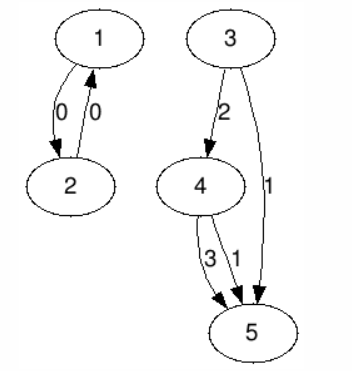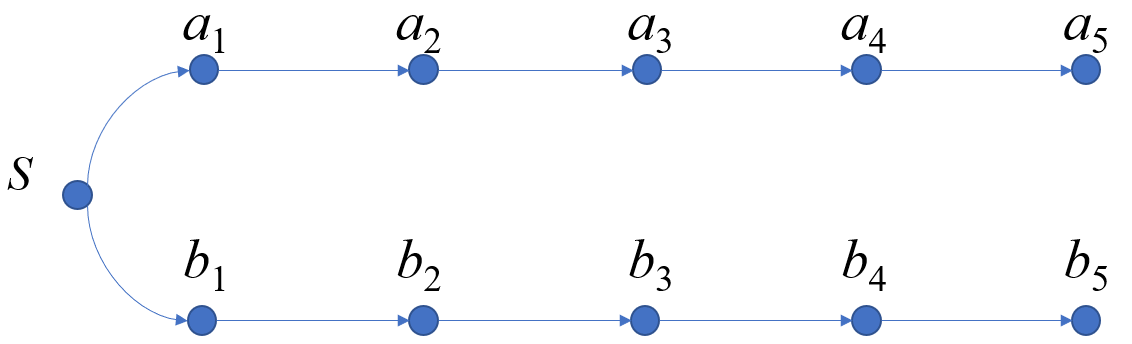# 牛客 178A 最长路 题解【拓扑排序】【贪心】【分层图】【哈希】【倍增】

这个题是倍增+hash，细节比较多……

## 输入输出样例

5 6
1 2 0
2 1 0
3 4 2
4 5 3
4 5 1
3 5 1

Infinity
Infinity
899
29
0

## 说明## 说明

• $1\le n\le 1000000$
• $1 \le m\le 1000000$
• $0 \le$字符$\le 10^9$

 测试点编号 $n$ $m$ 特殊性质 $1,2,3,4$ $\le 1000$ $\le 1000$ $5,6$ 所有字符$=0$ $7,8$ 所有字符相等 $9,10,11,12,13,14,15,16$ 所有字符互不相等 $17,18$ $\le 200000$ $\le 200000$ $19,20$

## 题解：

这题关键在于求最长路，这个我们反向建图跑拓扑排序即可，不过没有必要跑tarjan，拓扑排序中没有进队的就在环上了。单纯考虑有向图最长路，直接用len[i]表示一下，拓扑排序转移。

难点在于输出路径并保证字典序最小。首先我们想到了一个贪心，就是每次转移边权最小的那个。但是这样在原图的后继出现同种字符时会有问题，因为无法存后面的状态，而用于存路径的答案值也是会被取模的，这里会出现小问题。我们对部分分进行分析可以发现，如果就按照这样做下去，可以拿到$3\sim 5$子任务的$60$分（正解写挂了也是$60$23333..），性价比极高。

但是我们还是要研究一下正解。正解的其中一个是比较好想的，倍增+哈希，但是很难实现。哈希是题目自带的，可以直接用（不怕被卡吗…），我们可以通过倍增来找多个序列后面第一条互不相等的边，这里跳倍增的过程中用哈希判一下前面一段是否相等，如果相等则继续跳，如果不等则减小到原来的$\frac 12$继续倍..减？但是有一点需要注意，这里每个点的数量级都不一样，因此需要互相转化，比如下面这个图：我们当发现$S\rightarrow a_1$与$S\rightarrow b_1$边权相等时，就要进行倍增，那么我们要比较接下来$2^i$条边是否全部相等怎么办？当我们做到$S$时，后面的这$10$个节点一定都已经做完了，那么后面的节点的答案值，也就是哈希值是都有了的，我们接下来分析怎么判断一串节点的边是否相等。

假设我们当前枚举到$i=1$，那么从下标为$1$的位置倍增$2^i$条边到了$3$，我们把数量级$29$用$p$来表示，则$hash_{a_1}=a_1\cdot p+a_2\cdot p^2+a_3\cdot p^3+a_4\cdot p^4+a_5\cdot p^5$，$hash_{b_1}$同理。同时$hash_{a_3}=a_3\cdot p+a_4\cdot p^2+a_5\cdot p^3$

这里肯定不能直接拿$hash_{a_3}$去减$hash_{a_1}$，因为数量级不一致，后面的不同会有影响。为了把两个式子拉到同一数量级，我们把$hash_{a_3}$乘上$p^2$，即把数量级小的统一乘上这个商值，分别把两式相减得到$hash_{a_1}-hash_{a_3}\cdot p^2,hash_{b-1}-hash_{b_3}\cdot p^2$就可以判断$a_1\cdot p+a_2\cdot p^2$与$b_1\cdot p+b_2\cdot p^2$是否相等了。

倍增的时候注意减去的是当前倍增区间，也就是长为$2^i$的那个，不是到$S$的距离。差的数量级数也是$2^i$，这里用快速幂完成就可以了，貌似多了个大约$\log n$的常数，可以预处理$29$的$1000000$以内的幂，不过给了3秒，卡过去了。时间复杂度$O(n\log n)$。

正解的另一种做法是做DP，首先把所有点的最长路求出来，然后按最长路长度分层，注意涉及到Infinity的点最好不要放进去。然后对连接每一层的边排序，第一关键字是边权，第二关键字是上一层点的被转移的时间，均为升序。然后从小到大枚举最长路长度，接着对每种长度枚举边，碰到还没有被转移的就转移。这样贪心地让最优的情况转移，而且用了两个关键字，目的是使得第一关键字相同的先转移后面更优的，主要是控制指向同一个点的边的转移顺序。这个思路虽说不好想，但是还是非常自然的，并且常数小，编程难度也小，算法一我写了一下午，这个做法只写了十来分钟…，需要多接触这种模型。

## Code of 算法一：

#include<cstdio>
#include<cstring>
struct edge
{
int n,nxt,v;
edge(int n,int nxt,int v)
{
this->n=n;
this->nxt=nxt;
this->v=v;
}
edge(){}
}e;
{
}
long long hs;
int f,len;
int in,pre;
int q,l=0,r=0;
long long qpow(long long x,long long y)
{
long long ans=1,m=x;
while(y)
{
if(y&1)
ans=ans*m%998244353;
m=m*m%998244353;
y>>=1;
}
return ans;
}
int main()
{
int n,m,u,v,w;
scanf("%d%d",&n,&m);
for(int i=1;i<=m;++i)
{
scanf("%d%d%d",&u,&v,&w);
++in[u];
}
for(int i=1;i<=n;++i)
if(!in[i])
{
for(int j=0;j<=20;++j)//“汇点”需要倍增预处理到自己
f[j][i]=i;
q[++r]=i;
}
while(l<r)
{
int x=q[++l];
{
v=e[i].n;
--in[v];
if(!in[v])
q[++r]=v;
if(len[x]+1>len[v])
{
f[v]=x;
pre[v]=e[i].v;//其实这几行重复了几遍，可以写在最后或者写个函数的...
hs[v]=(hs[x]+e[i].v)*29%998244353;//转移
len[v]=len[x]+1;
}
else if(len[x]+1==len[v])
{
if(e[i].v<pre[v])
{
f[v]=x;
pre[v]=e[i].v;
hs[v]=(hs[x]+e[i].v)*29%998244353;
}
else if(e[i].v==pre[v])
{
int tx=x;
int y=f[v];
for(int j=20;j>=0;--j)
if((hs[f[j][tx]]*qpow(29,1<<j)%998244353-hs[tx]+998244353)%998244353==(hs[f[j][y]]*qpow(29,1<<j)%998244353-hs[y]+998244353)%998244353)
{
tx=f[j][tx];
y=f[j][y];
}
if(pre[tx]<pre[y])
{
f[v]=x;
pre[v]=e[i].v;
hs[v]=(hs[x]+e[i].v)*29%998244353;
}
}
}
for(int j=1;j<=20;++j)
f[j][v]=f[j-1][f[j-1][v]];
}
}
for(int i=1;i<=n;++i)
if(in[i])
puts("Infinity");
else
printf("%lld\n",hs[i]);
return 0;
}

## Code of 算法二：

#include<cstdio>
#include<cstring>
#include<vector>
#include<algorithm>
using std::max;
using std::sort;
using std::vector;
struct edge
{
int n,nxt,v;
edge(int n,int nxt,int v)
{
this->n=n;
this->nxt=nxt;
this->v=v;
}
edge(){}
}e;
{
}
int f,rk,cnt=0;//存的是最长路条数和转移顺序
struct Edge
{
int from,to,v;
friend bool operator <(Edge a,Edge b)
{
if(a.v!=b.v)
return a.v<b.v;
return rk[a.from]<rk[b.from];
}
Edge(int from,int to,int v)
{
this->from=from;
this->to=to;
this->v=v;
}
Edge(){}
};
vector<Edge> g;
int in,q,l=0,r=0;
long long ans;
int main()
{
freopen("data.in","r",stdin);
freopen("ans.out","w",stdout);
int n,m,u,v,w;
scanf("%d%d",&n,&m);
for(int i=1;i<=m;++i)
{
scanf("%d%d%d",&u,&v,&w);
++in[u];
}
for(int i=1;i<=n;++i)
if(!in[i])
q[++r]=i;
int mx=0;
while(l<r)
{
int x=q[++l];
{
f[e[i].n]=max(f[e[i].n],f[x]+1);
mx=max(f[e[i].n],mx);
--in[e[i].n];
if(!in[e[i].n])
q[++r]=e[i].n;
}
}
for(int i=1;i<=n;++i)
if(!in[i])
if(f[e[j].n]==f[i]+1)
g[f[i]].push_back(Edge(i,e[j].n,e[j].v));
for(int i=0;i<=mx;++i)
{
sort(g[i].begin(),g[i].end());//对边排序
for(vector<Edge>::iterator it=g[i].begin();it!=g[i].end();++it)
if(!rk[it->to])
{
rk[it->to]=++cnt;
ans[it->to]=(ans[it->from]+it->v)*29%998244353;//正常转移
}
}
for(int i=1;i<=n;++i)
if(in[i])
puts("Infinity");
else
printf("%lld\n",ans[i]);
return 0;
}

### 说点什么Subscribe

/* */